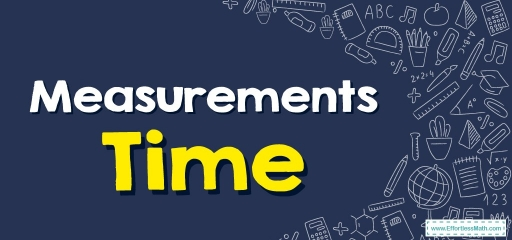# Measurements – TimeTime can be defined as a period in which a particular event occurs, has occurred, or will occur. It’s a measurable quantity, plus it’s additionally infinite.

Time gets measured via seconds, minutes, and hours, as well as days, months, and years.

There are a lot of additional tinier units of time like milliseconds or microseconds. The tiniest time unit is called a ‘Zeptosecond’.

An hour equals $$60$$ minutes, plus a minute equals $$60$$ seconds.

The majority of analog clocks have $$3$$ sticks mounted in their center which spins all around the clock so you can tell what time it is. They are known as clock hands. Those $$3$$ hands get used for telling hours, minutes, and seconds.

## The Hour hand

The small hand is the hour hand. This hand lets one know what hour it is. Whenever the hour hand gets in-between $$2$$ numbers, it lets you know you are in between those $$2$$ hours. Then its shorthand or hour hand goes around $$1$$ whole rotation around the clock dial in twelve hours.

## The Minute hand

The clock’s longer hand is known as the minute hand. The clock’s longhand or minute hand goes around the circular dial once in $$1$$ hour.

## The Second hand

The extremely thin hand which is normally the same size as a minute hand is called a second hand. This hand goes $$1$$ time around the clock dials in $$1$$ minute and signals the seconds for each minute.

Time measures for fifteen-thirty or forty-five minutes can be shown this way:

Quarter to – That implies fifteen minutes are remaining to carry out the given hour. For instance, $$04$$:$$45$$ implies, there are fifteen minutes to get to $$5$$:$$00$$. That’s read as “a quarter to six”.

Quarter past – That implies fifteen minutes have gone by after the hour has begun. For instance, $$06$$:$$15$$ entails, fifteen minutes have gone after $$6$$:$$00$$. That gets read as “a quarter past seven”.

Half-past – This represents thirty minutes that have gone by after the hour has begun. For instance, $$03$$:$$30$$ indicates, thirty minutes have gone by after $$3$$:$$00$$. That gets read as “a half-past eight”.

## A.M. and P.M.

• Time going from $$12$$:$$00$$ in the midnight to $$11$$:$$59$$ in the morning is described as ‘a.m’.
• Time going from $$12$$:$$00$$ in the afternoon to late night $$11$$:$$59$$ is described as ‘p.m’.

## 12-hour and 24-hour clocks

• With a $$12$$-hour time format, the time gets expressed via a.m or p.m. So, $$8$$:$$20$$ in the morning is shown as $$8$$:$$20$$ a.m. The equivalent time in the evenings is shown as $$8$$:$$20$$ p.m.
• With a $$24$$-hour time format, $$6$$:$$30$$ a.m. gets shown as $$6$$:$$30$$ hours, but $$6$$:$$30$$ p.m. is shown as $$18$$:$$30$$ hours. This is done by adding $$12$$ to the hour number. Though, the minute number stays the same.

### Measurements – Time – Example 1:

How much time has passed?

$$3:40$$ AM to $$5:20$$ AM:____ hours and____ minutes.

Solution:

We know that every hour is $$60$$ minutes. From $$3:40$$ AM to $$5:20$$ AM, $$100$$ minutes have passed, that is, $$1$$ hour and $$40$$ minutes.

### Measurements – Time – Example 2:

Solve

There are_____ second in $$12$$ minutes.

$$1$$ minute $$= 60$$ seconds. So $$12$$ minutes $$= 12 × 60=720$$. There are $$760$$ seconds in $$12$$ minutes.

## Exercises for Measurements – Time

Solve

1. $$2:50$$ AM to $$4:20$$ AM:____ hours and____ minutes.
2. $$3:15$$ PM to $$5:30$$ PM:____ hours and____ minutes.
3. There are____ minutes in $$20$$ hours.
4. There are____ second in $$15$$ minutes.
1. $$1$$ hour and $$30$$ minutes.
2. $$2$$ hour and $$15$$ minutes.
3. $$1,200$$
4. $$900$$

### What people say about "Measurements – Time - Effortless Math: We Help Students Learn to LOVE Mathematics"?

No one replied yet.

X
30% OFF

Limited time only!

Save Over 30%

SAVE $5 It was$16.99 now it is \$11.99